Analyze performance characteristics of pulsed, frequency-modulated, and phase-coded waveforms

## Description

The Radar Waveform Analyzer app lets you explore the properties of signals commonly used in radar. You can display 2-D plots and 3-D images that let you visualize waveform time series and spectra.

The app lets you change waveform parameters and see how different parameter values affect the appearance and properties of the waveform. Waveform parameters include pulse repetition frequency (PRF), pulse duration, and bandwidth. The app displays basic waveform characteristics such as range resolution, Doppler resolution, and maximum range. When you launch the app, the Real and Imaginary and Spectrum tabs are shown by default. You can simultaneously overlay plots of multiple waveforms.

You can select different types of displays using this pull-down menu.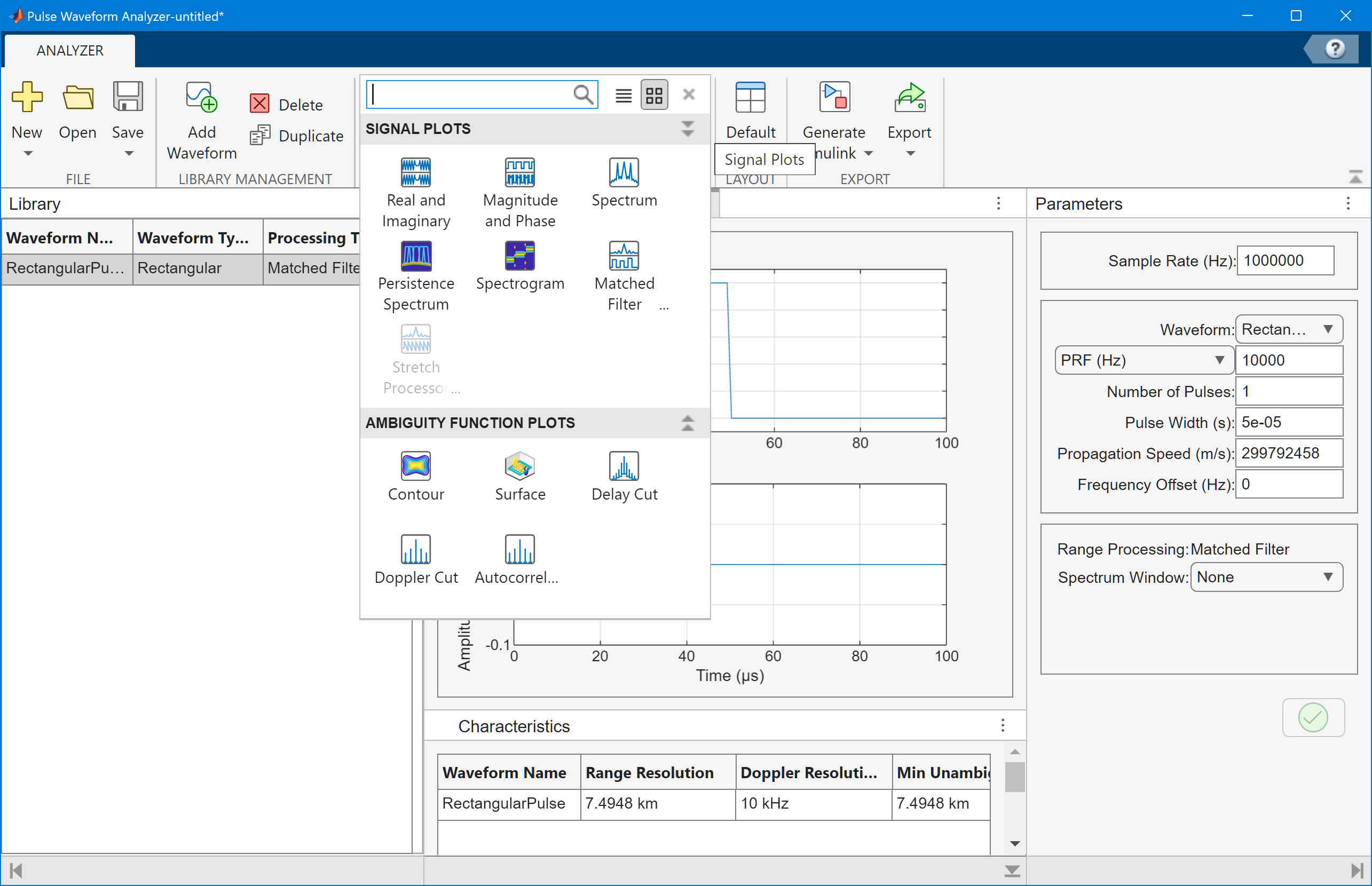The app lets you analyze these types of waveforms:

• Rectangular

• Linear frequency modulation (LFM)

• Stepped FM

• Phase-coded waveforms

• Frequency modulation constant waveform (FMCW)

You can export waveforms as workspace variables or files containing:

You can also create waveform blocks and Pulse Waveform Library blocks for use in Simulink®.

You can also use this app for sonar applications by choosing the appropriate propagation speed.

## Open the Radar Waveform Analyzer App

• MATLAB® toolstrip: On the Apps tab, under Signal Processing and Communications, select the app icon, or

• MATLAB command prompt: Enter `radarWaveformAnalyzer`. For ways to use the app programmatically, see Programmatic Use.

## Examples

expand all

This example shows how to analyze a rectangular waveform. An ideal rectangular waveform jumps instantaneously to a constant value and stays there for some duration.

When you open the app, the Library tab shows the default rectangular waveform and the center panel displays the waveform shape or spectrum. First, set the Sample Rate (Hz) to 3 MHz. The same sample rate applies to all waveforms that you analyze.

You can rename the waveform by right-clicking its name. For example, change the name to `RectangularPulse`.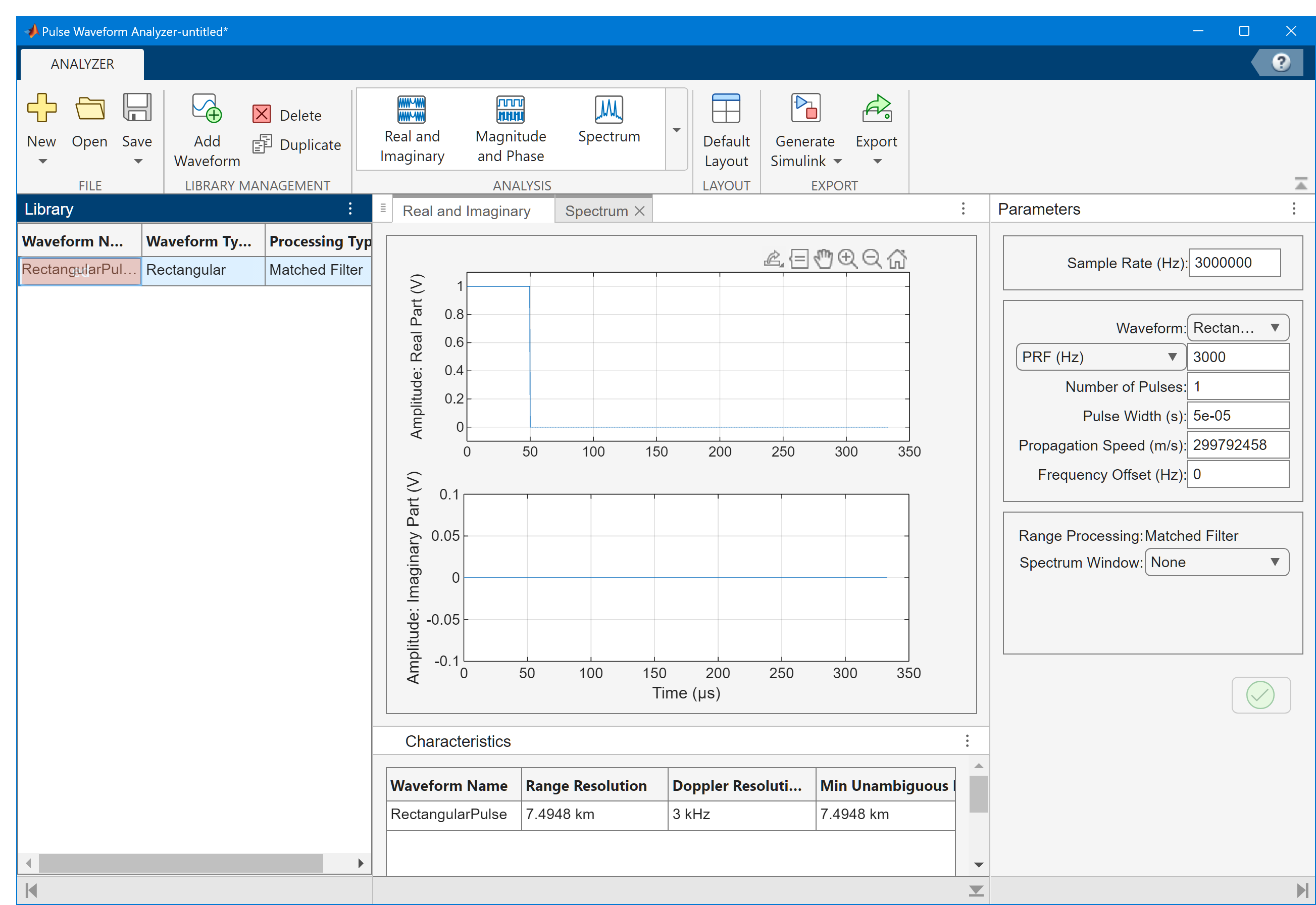Design the pulse for a maximum range of 50 km. For this range, the time for a signal to propagate and return is 333 μs. Therefore, allow 333 μsec between pulses, equivalent to a pulse repetition frequency (PRF) of 3000 Hz.

Set the Pulse Width to 50 μs.

Change the value of the speed of light in the Propagation Speed field to a more precise value by entering `physconst('Lightspeed')`. You can use workspace variables and MATLAB functions in any editable field.

After you select the green check mark, the app displays a range resolution of approximately 7.5 km in the Characteristics tab on the right hand panel. In this panel, you can scroll right to see other properties. The range resolution of a rectangular pulse is roughly 1/2 the pulse-width multiplied by the speed of light. The Doppler resolution is approximately the width of the Fourier transform of the pulse.

In the center panel of the window select the Real and Imaginary tab to plot the waveform.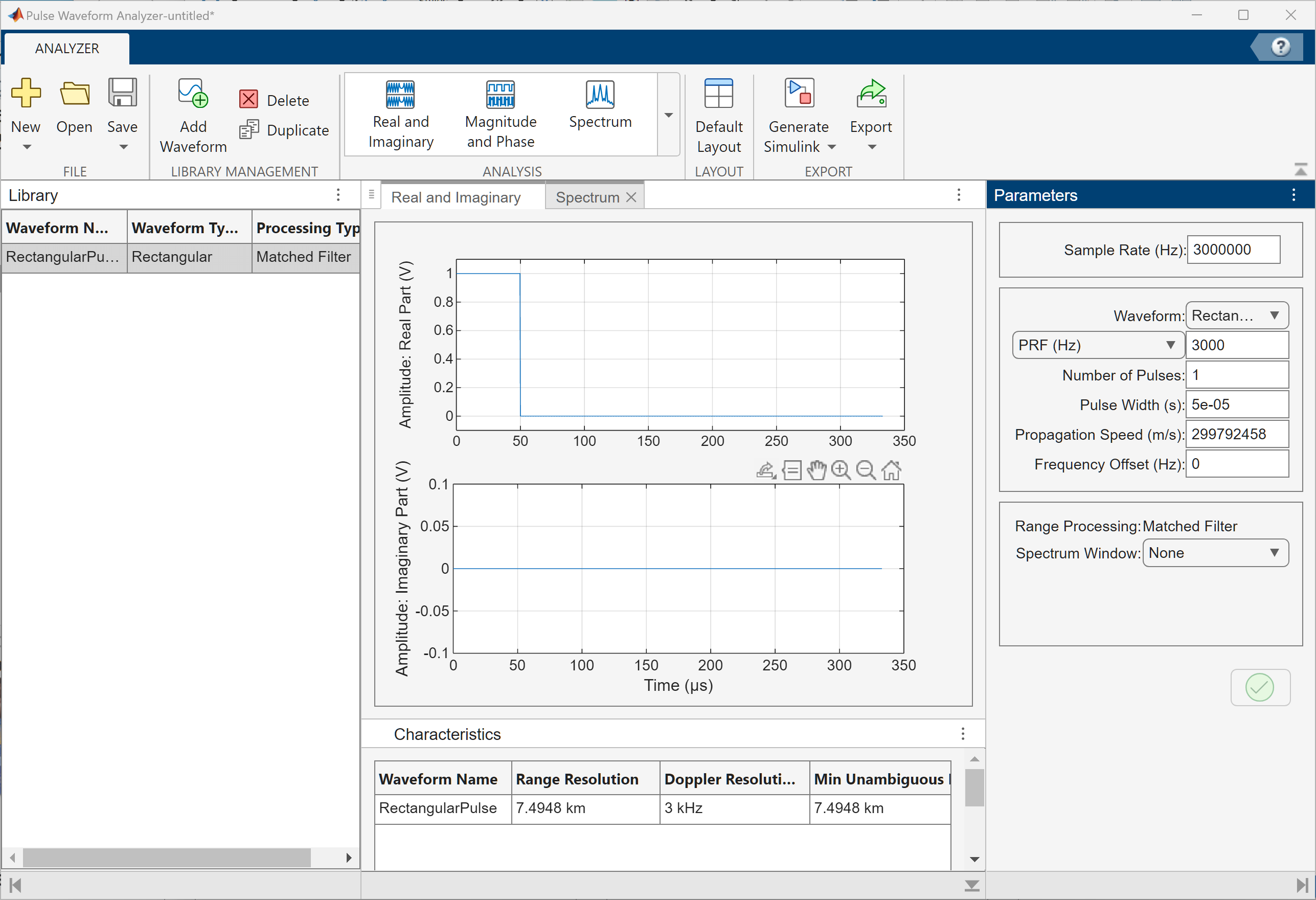Select the Spectrum tab In the center panel of the window to show the power spectral density.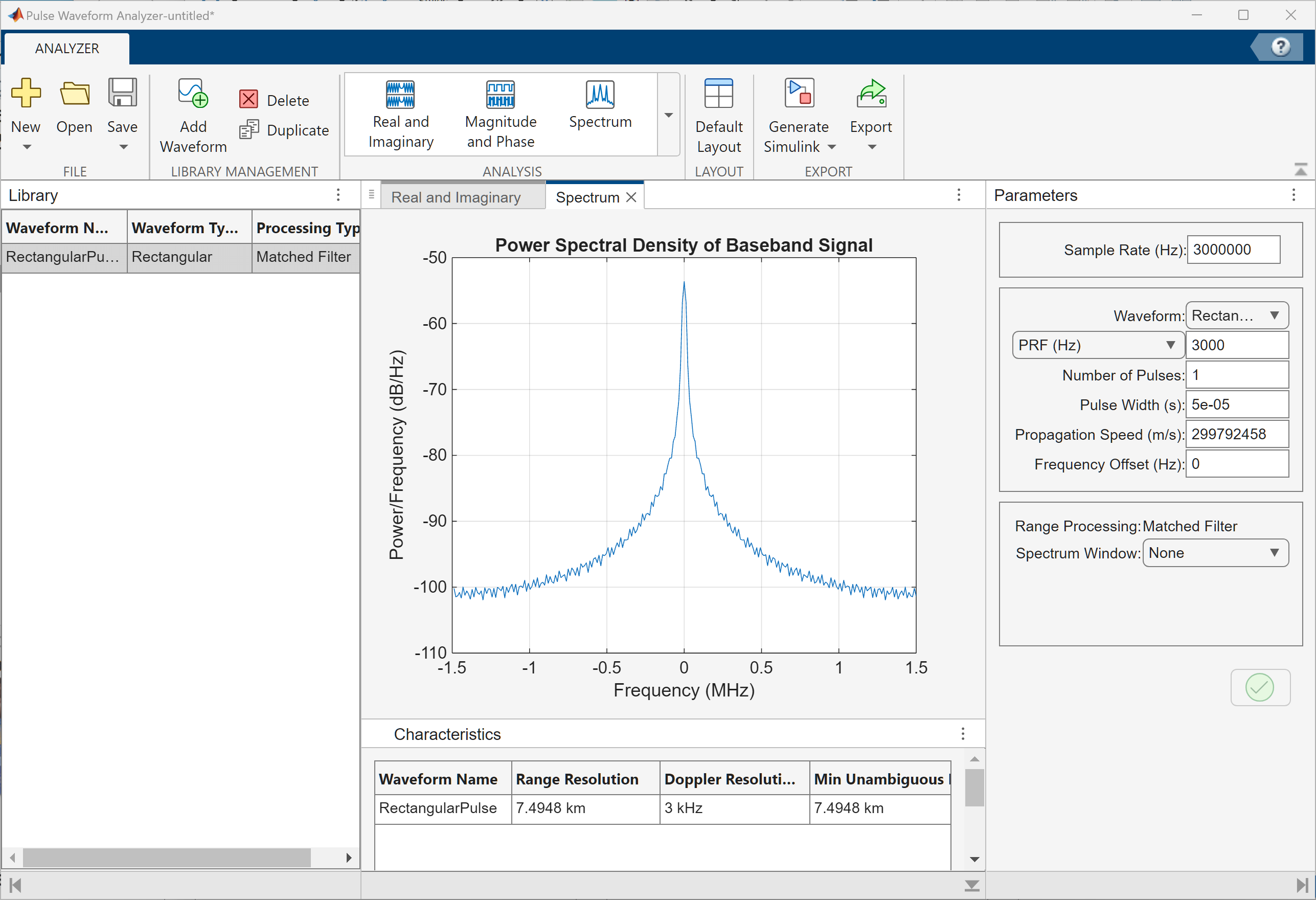You can display the joint range-Doppler resolution by selecting Surface from the Ambiguity Plots menu.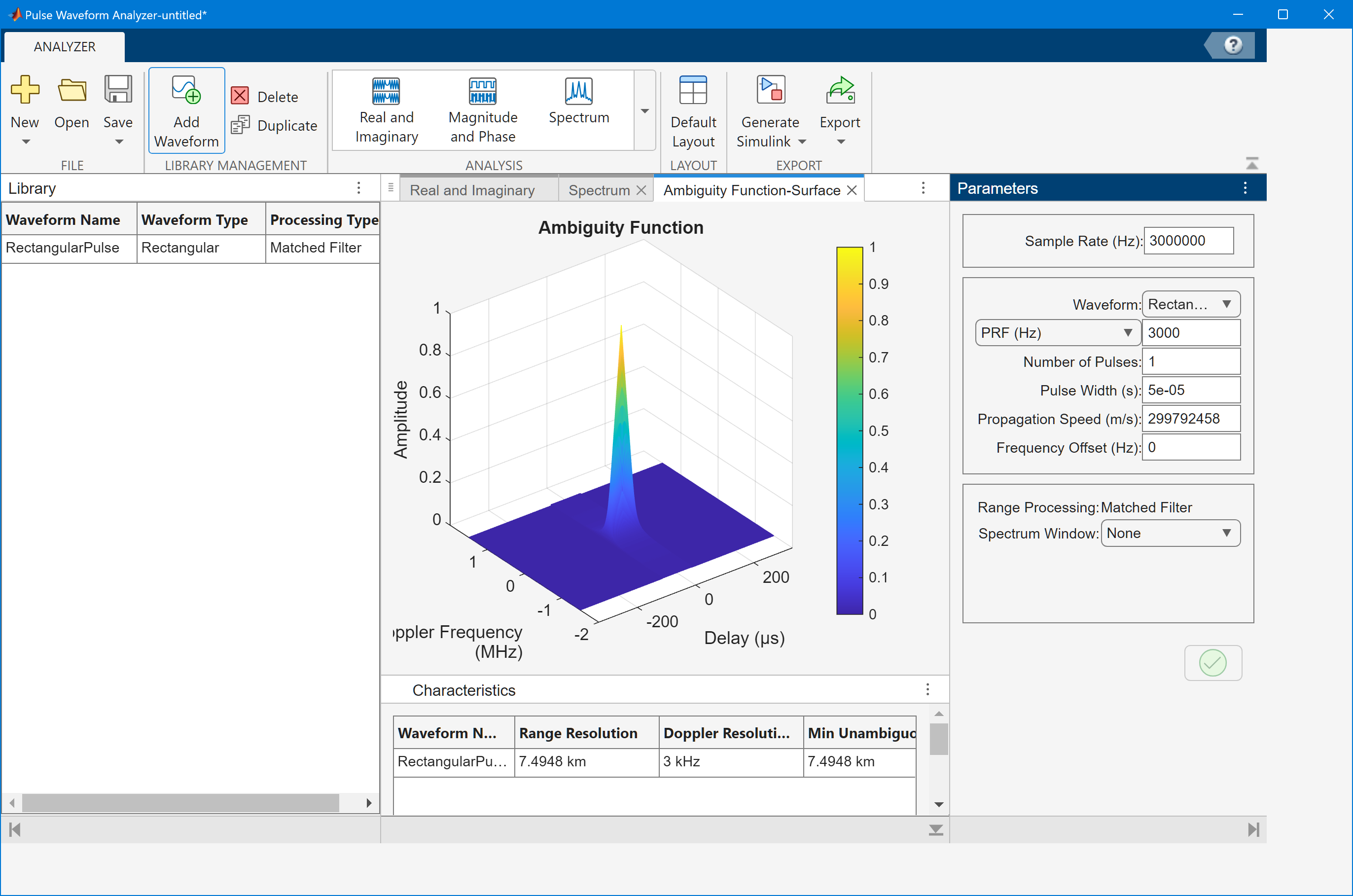This example shows how to improve range resolution using a linear FM waveform. In the previous example, the range resolution of the rectangular pulse was poor, approximately 7.5 km. You can improve the range resolution by choosing a signal with a larger bandwidth. A good choice is a linear FM pulse.

In the Parameter tab, change the Waveform to `Linear FM`. Then, for example, change the waveform name to `LinearFMWaveform`. This type of pulse has a varying frequency which can either increase or decrease as a linear function of time. Keep the sample rate at 3 MHz.

Choose the Sweep Direction as `Up`, and the Sweep Bandwidth as 1 MHz.

You can see that keeping the same pulse width as in the previous example, improves the range resolution to 150 m, as shown in the Characteristics tab.While the range resolution becomes better, the Doppler resolution is worse than the resolution of a rectangular waveform. You can see this by selecting the Surface ambiguity plot. The Ambiguity Function-Surface tab shows this tradeoff between Doppler resolution and range resolution.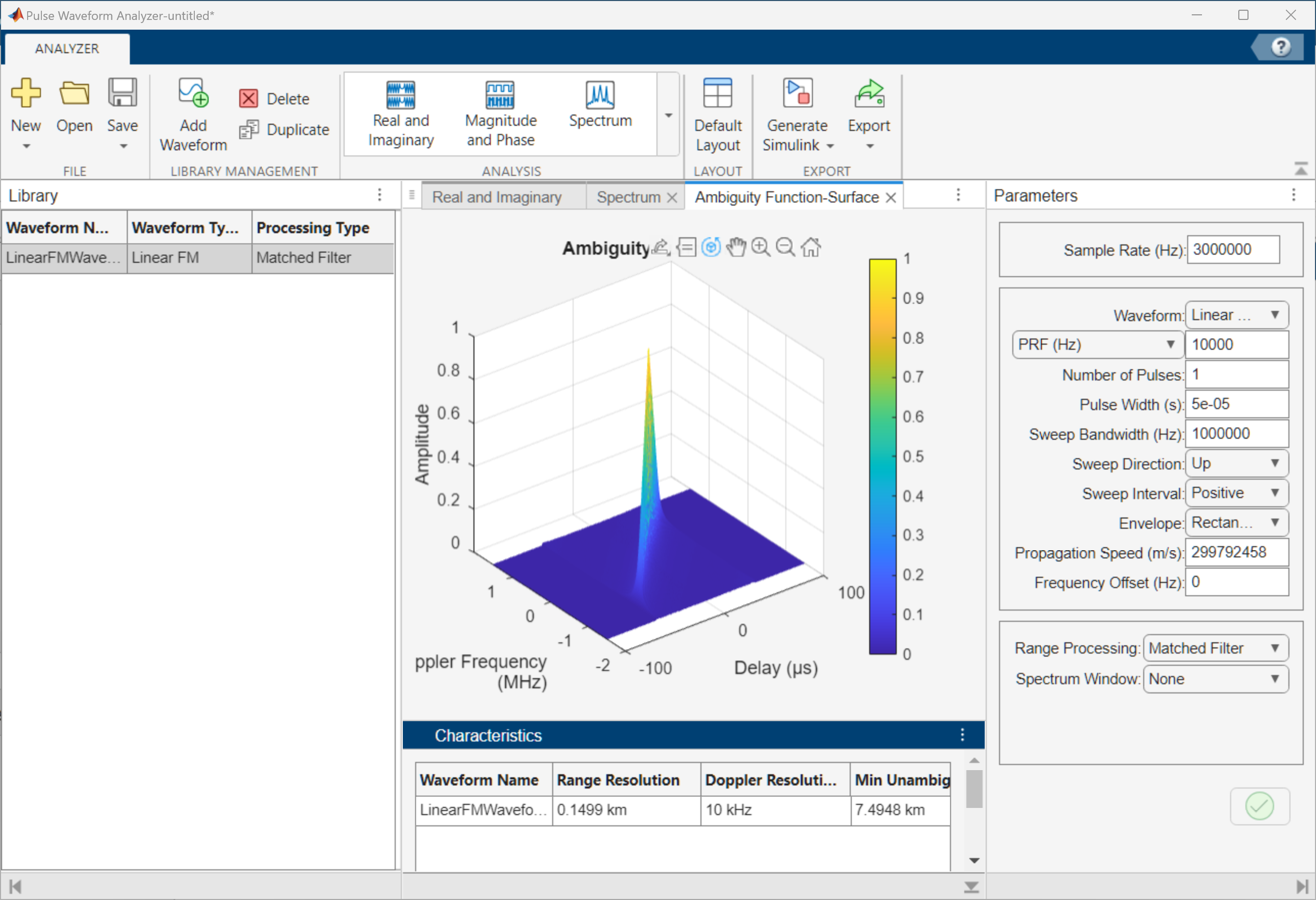This example shows how to display the spectrogram of a linear FM waveform with and without frequency reassignment.

Use the same signal parameters as in the previous example.

Select Spectrogram from the Signal Plots drop-down menu. Then, select the Reassigned check box to show the frequency reassigned spectrogram (reassignment is turned on by default). Set the Threshold to -100 dB. Frequency reassignment is a technique for sharpening the magnitude spectrogram of a signal using information from its phase spectrum. For more information on frequency reassignment, see Fulop and Kelly (2006) .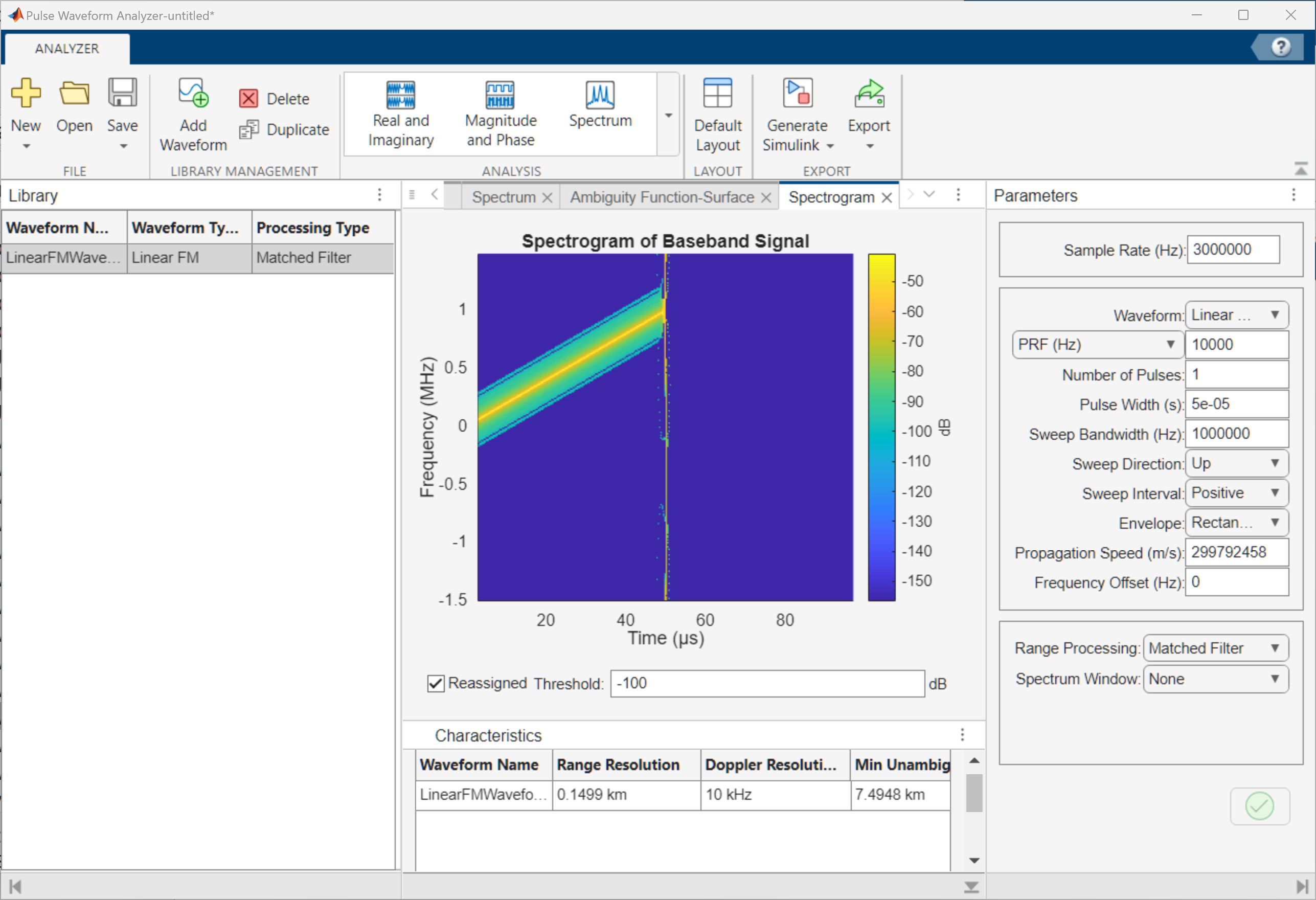You can vary the Threshold setting to show or hide weaker spectrum components.

To view the conventional spectrogram, clear the Reassigned check box.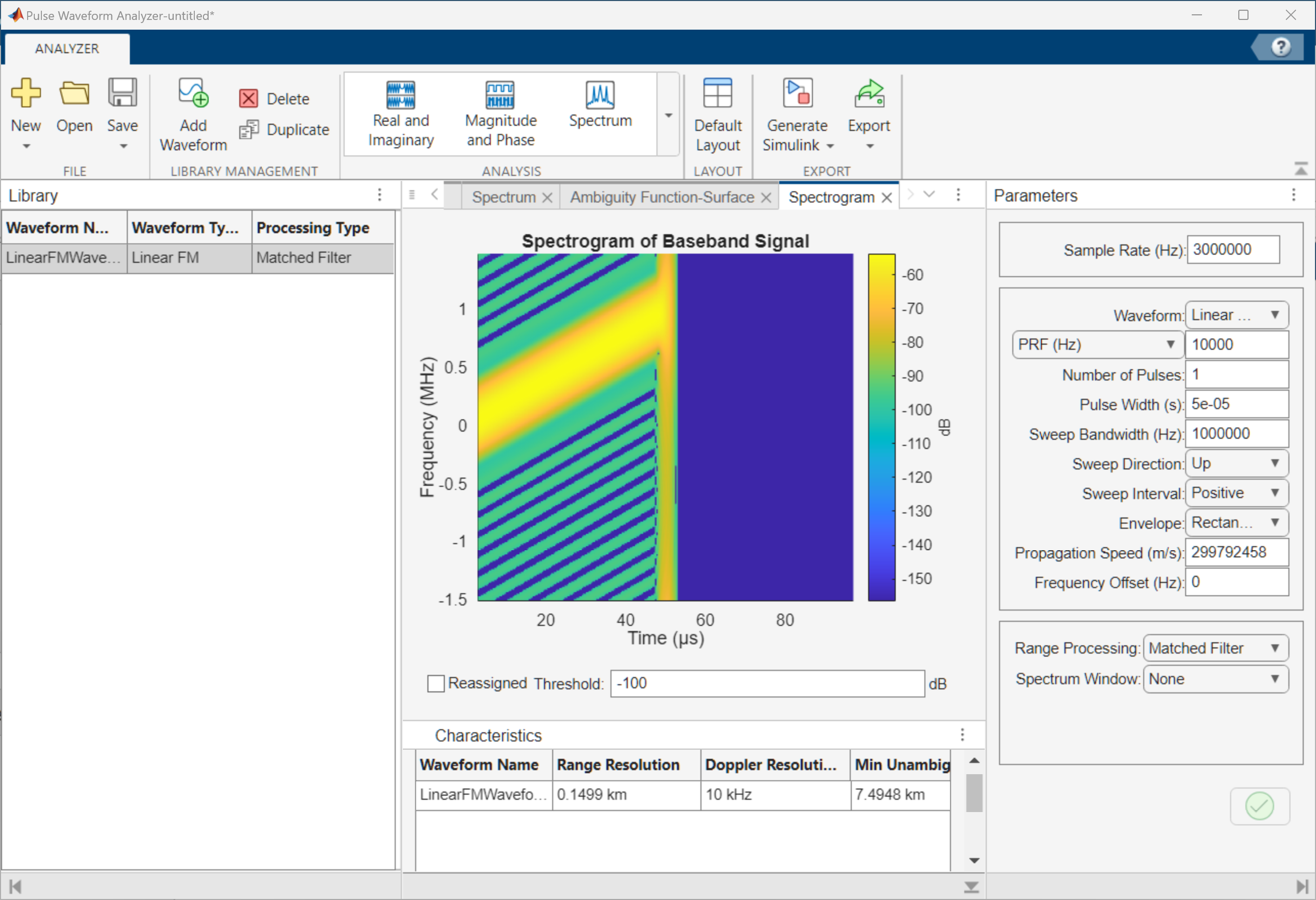Again, you can vary the Threshold Value setting to show or hide weaker spectrum components.

This example shows how to display the two signals simultaneously.

First, create a rectangular waveform having the same parameters as used in the first example. Then, rename the waveform to `RectangularPulse`.

Next, create an LFM waveform. Click the Add Waveform button. Rename the second waveform to `LinearFMPulse`. Set the waveform parameters to the same values as in the second example.

Select both waveforms in the Library panel. The displays now shows the waveforms, spectra, and characteristics for both waveforms.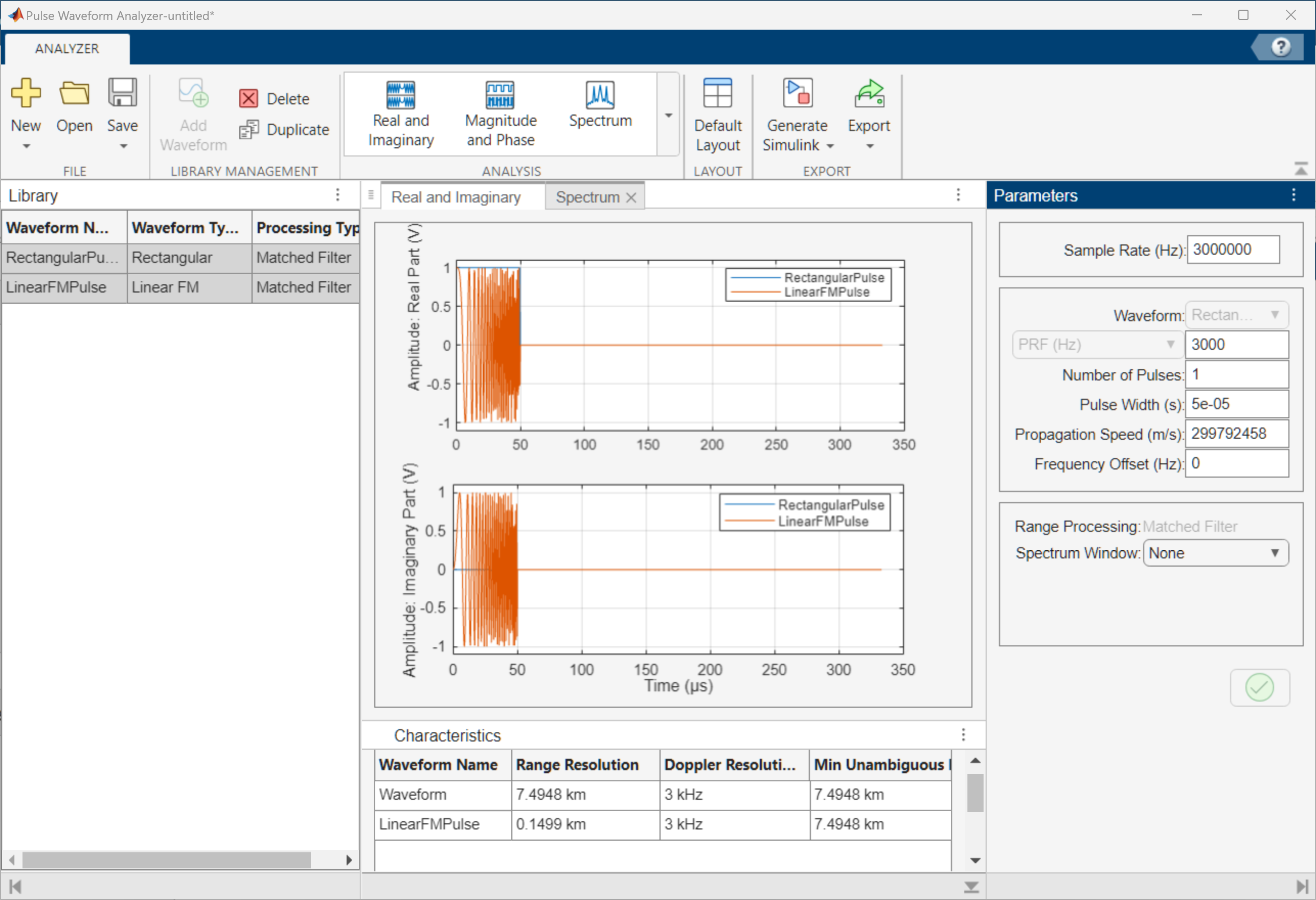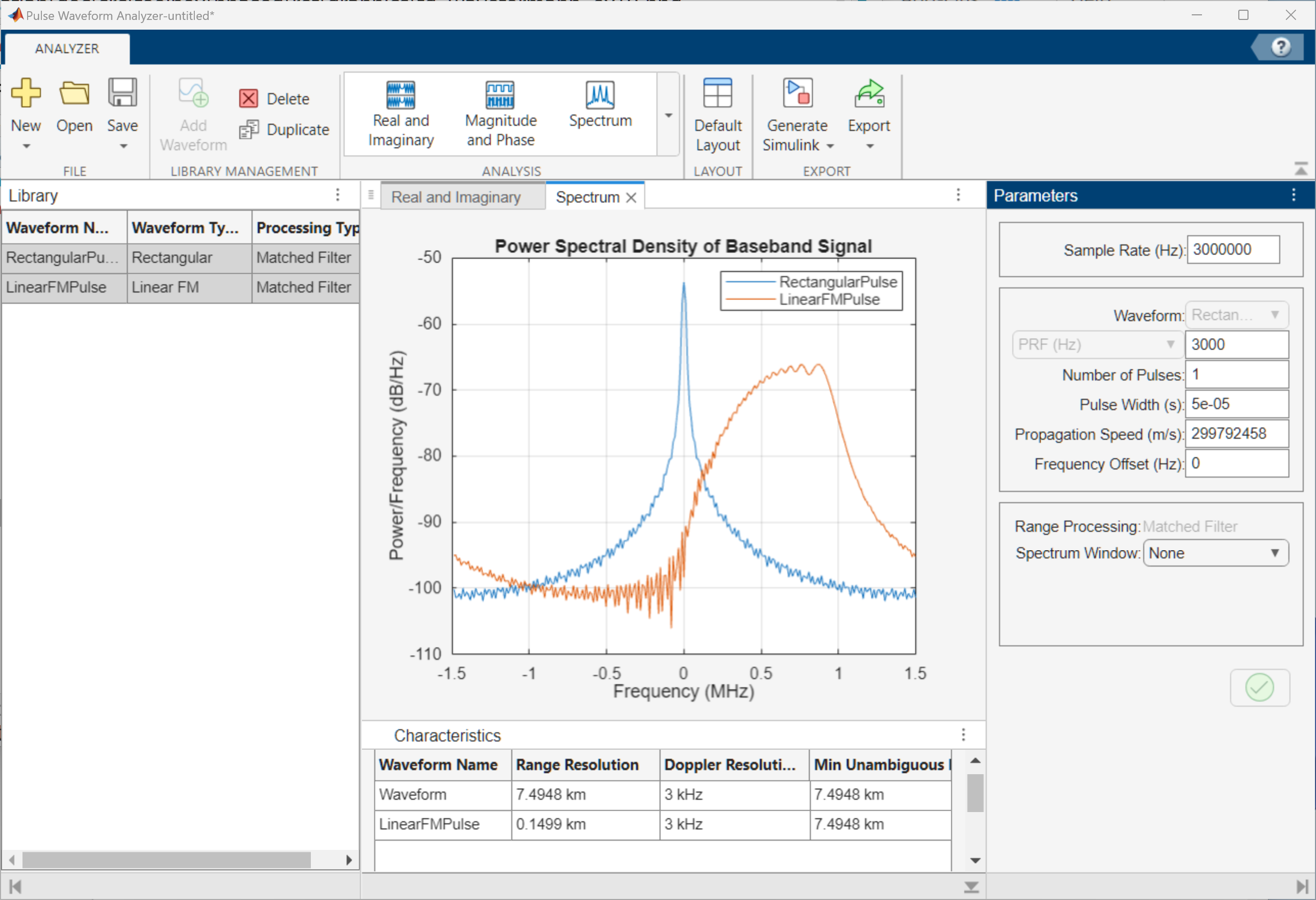## Programmatic Use

expand all

You can run radarWaveformAnalyzer from the command line.

`radarWaveformAnalyzer(wav)` opens the Radar Waveform Analyzer app and imports and plots the waveform `wav`. `wav` can be a variable in the workspace representing a waveform object such as:

```wav = phased.LinearFMWaveform('SampleRate',fs, ... 'SweepBandwidth',200e3,... 'PulseWidth',1e-3,'PRF',1e3); radarWaveformAnalyzer(wav)```
or you can enter the object directly:
```radarWaveformAnalyzer(phased.LinearFMWaveform( ... 'SampleRate',fs, ... 'SweepBandwidth',200e3,... 'PulseWidth',1e-3,'PRF',1e3))```

`radarWaveformAnalyzer(wavlib)` opens the Radar Waveform Analyzer app and imports a `phased.PulseWaveformLibrary` object, `wavlib`. For example, construct the waveform library object from three waveforms with a common sample rate of 1 MHz. Then run from the command line:

```waveform1 = {'Rectangular','PRF',1e4,'PulseWidth', 50e-6}; waveform2 = {'LinearFM','PRF',1e4,'PulseWidth',50e-6, ... 'SweepBandwidth',1e5,'SweepDirection','Up',... 'SweepInterval', 'Positive'}; waveform3 = {'PhaseCoded','PRF',1e4,'Code','Zadoff-Chu', ... 'SequenceIndex',3,'ChipWidth',5e-6,'NumChips',8}; fs = 1e6; wavlib = phased.PulseWaveformLibrary('SampleRate',fs, ... 'WaveformSpecification',{waveform1,waveform2,waveform3}); radarWaveformAnalyzer(wavlib)```

 Fulop, Sean A., and Kelly Fitz. "Algorithms for computing the time-corrected instantaneous frequency (reassigned) spectrogram, with applications." Journal of the Acoustical Society of America. Vol. 119, January 2006, pp. 360–371.